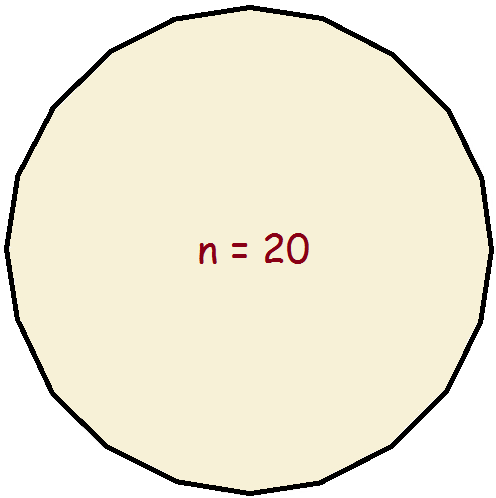Is a Circle a Polygon or Not? Implications for CalculusA 12-sided dodecagon. What do you think? Is the circle a polygon, or not?

As a result of watching a child’s video, I previously wrote a brief piece about the “corners” of a circle. The video was designed to teach children the various shapes—how many sides does a shape have, and how many corners?

The video maintained a circle has no corners. I called that into question. I still do. And yet, I do not. I now think it’s all in how you look at it. Or, you might say, it’s all in the mathematical perspective. Consider.

The Circle by Definition

One can define the two-dimensional circle as the complete collection or “set” of points equidistant from a set point, not part of the circle. Since it takes at least three points to create a corner, by derivation in this fashion, some say the circle has no corners. But is that the end of the discussion?

Circle a Polygon? By the Method of Limits

One can visualize a circle through the process of incrementally adding sides to a regular polygon. A triangle becomes a square; the square becomes a pentagon; the pentagon becomes a hexagon; the hexagon becomes a heptagon; the heptagon becomes an octagon; and so on.A 20-sided icosagon.

Even if it could be accomplished, the number of sides would be infinite, rather than zero. There are two ways around this that I can come up with. Option one: it could be declared that a true circle can never be derived by the method of limits. Is the circle a polygon? No. But consider again…

Such a supposition poses a problem: it suggests the method of limits must be abandoned in all cases. This would shut down what is undoubtedly the most important supposition of The Calculus. Rather than do that, perhaps it would be best to use option two. Ignore the whole issue.

Finally!

My final take on the matter is that mathematicians need to take stock on reality. Math is only a tool. It, like computers or even words, is not an end in itself, only a tool. To say a polygon of ever increasing sides never makes it to a true circle is ignorant. A circle is a polygon that has had its number of sides increased over time and has had that occur forever. It made it!

Note: You might also enjoy Point on a Line, a Line on a Plane, and a Plane in Space

References: ← Back to Math-Logic-Design
← Home

4 thoughts on “Is a Circle a Polygon or Not? Implications for Calculus”

• Allow me to suggest an alternate name for a circle — an infinigon. If that is the case, is not a cone then an infinigonal pyramid?

• Isaac Chan

I brought up a problem like this to a math teacher at my school and I created 2 proofs that can prove a circle is a polygon… kinda. So here they are:

Proof #1
1. Take (n-2)180/n and make n=∞, one internal angle would equal 180.
2. The formula above only works for polygons.
3. The only shape that could ever satisfy the needs of (∞ -2)180/∞ =180 is a circle.
4. Saying this, because a circle has 360 deg. then a circle is considered a 2-sided polygon.

Proof #2
1. To find the internal angle of a shape you must find the least amount of triangles that can fit in it. (ex. 2 in square, 3 in pentagon)
2. A square has 2 triangles so they add up to 360 deg.
3. This means a circle is not 360 deg.
4. Taking (∞ -2)180/∞ =180 into account, the circle is now an infinite pointed shape with infinite deg.

Tell me what you think because this stuff fascinates me.

• I’m not quite certain I am following Proof #2. But I really like Proof #1. Someone might try to claim (n-2)/n is not equal to 1 if n=∞, but I would disagree.

• I enjoyed reading this but my math is not good enough to comment on the technicalities. Loved the point about math being a TOOL! Yes!